# Additional Question Answers: Real Numbers Notes | Study Mathematics (Maths) Class 10 - Class 10

## Class 10: Additional Question Answers: Real Numbers Notes | Study Mathematics (Maths) Class 10 - Class 10

The document Additional Question Answers: Real Numbers Notes | Study Mathematics (Maths) Class 10 - Class 10 is a part of the Class 10 Course Mathematics (Maths) Class 10.
All you need of Class 10 at this link: Class 10

Q.1. State Euclid’s division algorithm.
Solution: Given two positive integers ‘a’ and ‘b’, there exists a unique pair of integers such that
a = (b × q) + r,  0 ≤ r < b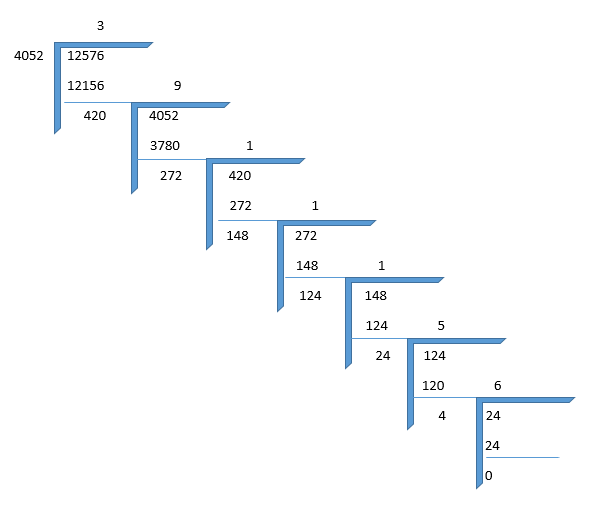This method is Euclid’s division algorithm

Q.2. State the fundamental theorem of arithmetic.
Solution: Every composite number can be expressed as the product of primes and this decomposition is unique apart from the order in which prime factors occur.

Q.3.Define an irrational number.
Solution: Those numbers which neither terminate in their decimal expansion nor can be expressed as recurring decimals are irrational numbers i.e., the numbers which cannot be expressed as p/q form (q ≠ 0), are called irrational numbers.

Q.4. Write the condition for a rational number which can have a terminating decimal expansion.
Solution: A rational number x = p/q can have a terminating decimal expansion if the prime factorisation of q is of the form of 2n · 5m, where m and n are non-negative integers.

Q.5. Write the condition for a rational number which has a non-terminating repeating decimal expansion.
Solution: A rational number x = p/q can have a non-terminating repeating decimal expansion if the prime factorisation of q is not of the form 2n · 5m, where n, m are non-negative integers.

Q.6. For any two integers, the product of the integers = the product of their HCF and LCM. Is this relation true for three or more integers?
Solution: No.

Q.7. If 6n is a number such that n is a natural number. Check whether there is any value of n ∈ N for which 6n is divisible by 7.
Solution: ∵ 6 = 2 × 3
∴  6n =  (2 × 3)n = 2n × 3n
i.e., the prime factorisation of 6n does not contain the prime number 7 thus the number 6n is not divisible by 7.

Q.8. Given that HCF (150, 100) = 50. Find LCM (150, 100).
Solution: ∵  LCM × HCF  = Product of the two numbers
∴  150 × 100 =  LCM × HCF
⇒ LCM × 50  =  150 × 100uy
⇒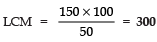Q.9. Given that LCM (26, 91) = 182. Find their HCF.
Solution: ∵  HCF × LCM  =  Product of the two numbers
∴   HCF × 182  =  26 × 91
⇒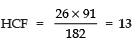Q.10. The LCM and HCF of the two numbers are 240 and 12 respectively. If one of the numbers is 60, then find the other number.
Solution: Let the required number be ‘x’.
∵  LCM × HCF   =  Product of the two numbers
∴ 60 × x  = 240 × 12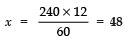Q.11. Write 98 as the product of its prime factors.
Solution:  ∵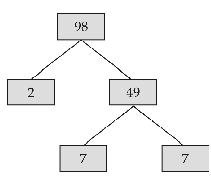∴ 98 = 2 × 7 × 7 ⇒  98 = 2 × 72

Q.12. Write the missing numbers in the following factorisation: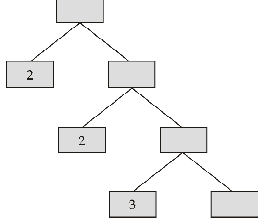Solution: ∵ 3 × 7 =  21,  21 × 2 = 42
and  42 × 2  = 84
∴ We have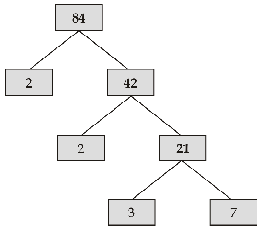Q.13. Without actually performing the long division, state whether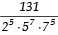will have a terminating or non-terminating repeating decimal expansion.
Solution: Let  =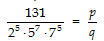∵ Prime factors of q are not of the for 2n · 5m.will have a non-terminating repeating decimal expansion.

Q.14. Without actually performing the long division, state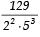will have a terminating or non-terminating repeating decimal expansion.
Solution: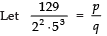∴ Prime factorisation of q is of the form  2n · 5mwill have a terminating decimal expansion.

Q.15. Without actually performing the long division, state whether 17/3125 will have a terminating decimal expansion or a non-terminating repeating decimal expansion.
Solution: ∵ The denominator of 17/3125 is given by
3125 =    5 × 5 × 5 × 5 × 5
=  1 × 55
= 2× 55    |∵ 20 = 1
∴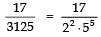i.e., 17/3125 is a terminating decimal.

Q.16. Express 156 as a product of its prime factors.
Solution:  ∵  156 = 2 × 78
= 2 × 2 × 39
=  2 × 2 × 3 × 13
∴ 156 = 22 × 3 × 13

Q.17. If product of two numbers is 20736 and their LCM is 384, find their HCF.
Solution: ∵  LCM × HCF = Product of two numbers
∴  384 × HCF =  20736
⇒ HCF  = 20736 /384   = 54.

Q.18. Find the LCM and HCF of 120 and 144 by Fundamental Theorem of Arithmetic.
Solution: We have 120 =  2 × 2 × 2 × 3 × 5 = 2× 3 × 5
144 =  2 × 2 × 2 × 2 × 3 × 3 = 24 × 32
∴ LCM  =  24 × 32 × 5 = 720
HCF  =  23 × 3 = 24

Q.19.Find the HCF × LCM for the numbers 100 and 190.
Solution:  HCF × LCM  =  1st Number × 2nd Number
=  100 × 190 = 19000.

Q.20. Find the (HCF × LCM) for the numbers 105 and 120.
Solution:  HCF × LCM =  1st number × 2nd number
= 105 × 120 = 12600.

Q.21. The decimal expansion of the rational number,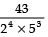will terminate after how many places of decimal?  (CBSE 2009)
Solution: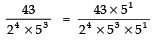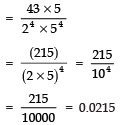Thus,will terminate after 4 places of decimal.

Q.22. Write whether the rational number will 51/1500 have a terminating decimal expansion or a non-terminating repeating decimal expansion.
Solution: We have, 51/1500  =  17/500
Prime factors of 500
=  2 × 2 × 5 × 5 × 5 =
22 × 53
which are in the form 2n · 5m
∴ It has a terminating decimal expansion.

Q.23. The HCF and LCM of the two numbers are 9 and 360 respectively. If one number is 45, write the other number.
Solution: ∵ Product of two numbers
=    LCM × HCF
∴ 45 × another number
= 9 × 360
⇒ another number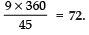Q.24. If  p/q is a rational number (q ≠ 0), what is condition of ‘q’ so that the decimal representation of p/q  is terminating?
Solution: The required condition is q = 2m × 5n, where m and n are whole numbers.

Q.25. Write a rational number between √2 and √3.
Solution:√2  =  1.41 ..... and
√3  =  1.73 .....
∴ one rational number between 1.41 .....and 1.73 ..... is 1.5
i.e., one rational number between √2 and √3 is 1.5.

Q.26. Complete the missing entries in the following factor-tree: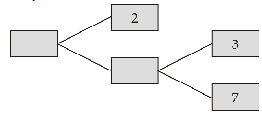Solution: ∵  3 × 7  =  21
and  21 × 2  = 42
∴ We have: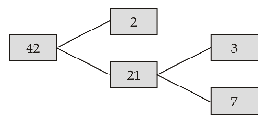Q.27. Write the HCF of the smallest composite number and the smallest prime number.
Solution:
∵ The smallest composite number = 4
= 2 × 2 = 22
The smallest prime number = 2
= 21
∴  HCF   =  2= 2

Q.28. Find the HCF of 960 and 432.
Solution: Applying Euclid’s Algorithm of division for 960 and 432, we have:
960 = 432 × 2 + 96  |96 ≠ 0
432 = 96 × 4 + 48  |48 ≠ 0
96 = 48 × 2 + 0
Since, the remainder  =  0
∴ HCF of 960 and 432 is 48

Q.29. Find the HCF of 72 and 120.
Solution:
Using Euclid’s algorithm of division for 72 and 120 we have,
120 = 72 × 1 + 48
72  = 48 × 1 + 24
48  =  24 × 2 + 0
∵ The remainder = 0
∴HCF (72, 120) is 24.

Q.30 What is the HCF of 52 and 130?
Solution: Using the Euclid’s algorithm of division for 52 and 130, we have:
130 =  52 × 2 + 26
52  =  26 × 2 + 0
∵ The remainder = 0
∴ HCF (130, 52) is 26.

The document Additional Question Answers: Real Numbers Notes | Study Mathematics (Maths) Class 10 - Class 10 is a part of the Class 10 Course Mathematics (Maths) Class 10.
All you need of Class 10 at this link: Class 10Use Code STAYHOME200 and get INR 200 additional OFF

## Mathematics (Maths) Class 10

58 videos|354 docs|138 tests

Track your progress, build streaks, highlight & save important lessons and more!

,

,

,

,

,

,

,

,

,

,

,

,

,

,

,

,

,

,

,

,

,

;